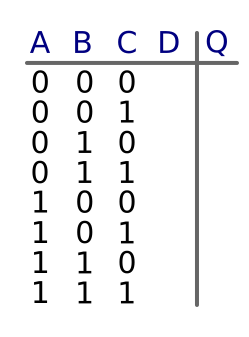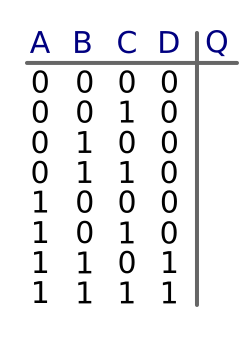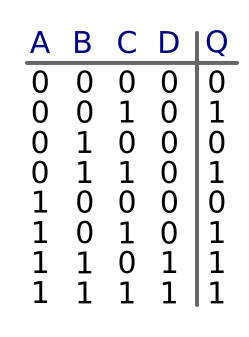# Combining gates

Martin McBride, 2017-01-16
Tags or gate and gate truth table
Categories logic logic gates

We can combine two or more gates to perform more complex functions.

## Combining gates

In this diagram, we have three inputs (A, B and C) and one output (Q). We are using an AND gate and an OR gate.We have also labelled the output of the AND gate as D. D is not an input, it is an intermediate value which we will use when calculating the truth table.

## Truth table

Since this circuit has 3 inputs, the truth table will have 8 rows. Here is the basic truth table, with space for D and Q to be added:D is equal to A AND B, so we can easily fill out the D column in the table. We just put 1 everywhere that A and B are both 1, and 0 everywhere else. Notice that D doesn't depend on C in any way:Q is equal to D OR C, so we can now fill out the Q column in the table - the actual output of the combination gate. We just put 1 everywhere that either D or C are 1, and 0 everywhere else:## Video

Copyright (c) Axlesoft Ltd 2021